# Full Stack Optimization of Transformer Inference: a Survey. Part 1 on Bottlenecks and Hardware Implications

tl;dr

The increasing compute and bandwidth demands for deploying recent Transformer models are posing a challenge in latency-sensitive applications. Therefore, there has been a focus on making Transformer models more efficient.

# A Paper Overview

## Abstract

Over the past few years, Transformer models have consistently demonstrated exceptional accuracy in various computer vision, natural language processing, and speech recognition tasks. Nevertheless, the increasing compute and bandwidth demands for deploying recent Transformer models are posing a challenge in latency-sensitive applications. Therefore, there has been a focus on making Transformer models more efficient, using different methods such as changing the architecture design and developing domain-specific accelerators.

The article surveys various approaches for efficient Transformer inference, including analysis of bottlenecks, hardware implications, optimization, and neural architecture search. The authors also perform a case study using Gemmini, an open-source deep neural network accelerator generator, and demonstrate that a full-stack co-design approach with the surveyed methods can yield significant speedups with minimal performance degradation for Transformer inference.

## Introduction

Deep learning models have become extremely large and require a significant amount of computing power, which has led to an interest in efficiently computing them on resource-constrained edge devices. CPUs and GPUs are commonly used, but their flexibility comes at the cost of reduced efficiency for deep learning tasks. Hardware accelerators have become popular due to their ability to perform a small number of distinct operations and leverage data reuse opportunities, leading to fast and efficient computation. In the past decade, both industry and academia have developed numerous enterprise deep learning accelerators that have been integrated into commodity hardware. Software frameworks and compilers have also improved to deploy various deep learning algorithms on accelerators, with mapping optimizations to improve performance and efficiency. However, evolving deep learning algorithms introduce new demands for hardware and software support, as well as their co-optimization, to satisfy deployment constraints.

The rise of Transformers and large language models for NLP tasks presents a new set of challenges for accelerator design and frameworks, with a focus on making Transformer inference more efficient due to their increasing size and complexity. This article discusses the challenges of designing efficient hardware and software solutions for running Transformer architectures, which are mostly composed of matrix multiplications (matmuls) together with memory-intensive nonlinear operations. In addition, Transformers have more complex computational graphs and dataflows compared to convolutional neural network (CNN) architectures.

The article also provides a survey and analysis of different approaches for efficient Transformer inference, including profiling runtime characteristics and bottlenecks, hardware architectures, optimization strategies, mapping and scheduling of operations, and neural architecture search. A case study applying these methodologies to Gemmini, a DNN accelerator generator, reveals key findings, including the importance of addressing the time spent on floating-point non-linear operations, the optimal accumulator and scratchpad sizes for Transformer accelerators, and the challenges of selecting appropriate scheduling decisions for Transformers.

The article also discusses the constraints imposed when fusing Layer Normalization with the preceding matmul in the Transformer architecture, and the need for further consideration in certain circumstances.

## Transformer Model Architecture and Performance Bottlenecks

#### High-Level Overview of Transformer Architecture

The Transformer architecture is composed of multiple Transformer blocks, each including a multi-head attention (MHA) module and a feed-forward (FFN) module, and each of which is followed by a Layer Normalization (LayerNorm) operation and a residual connection. The input sequence consists of l tokens, each represented by a vector of d dimension, forming a d x l matrix. The MHA module projects the sequence using query, key, and value weight matrices, this yields three different activations, namely the query, key, and value activations. The query, key, and value activations are then split into ℎ chunks, and forwarded to multiple attention heads, generating an activation matrix l x l, that is passed through Softmax and multiplied with the value chunk. The output is then concatenated and projected into the same dimension by a linear layer, passed through LayerNorm and added to a residual connection to get the MHA output.

In summary, an MHA module consists of six linear operations, four of which are identical weight-to-activation matmuls, and the remaining two of which are activation-to-activation matmuls. In thepaper, the authors refer to the first type of matmuls as projections and the second type of matmuls as activation-to-activation matmuls (act-to-act matmuls for short), as they have different run-time behaviors.

The FFN module consists of two linear layers and a non-linear layer in between, projecting the sequence from hidden dimension 𝑑 to higher dimension 𝑑(FNN) and back to the original dimension 𝑑.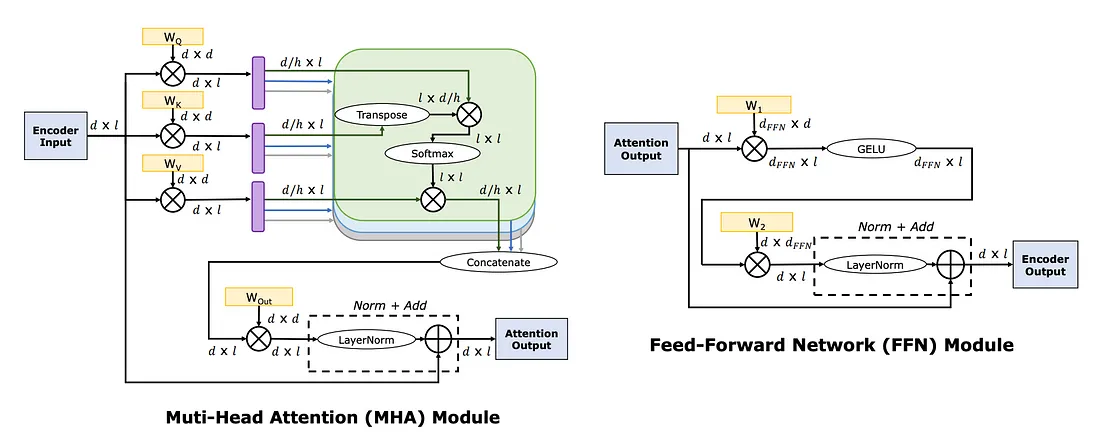Fig 1. Image taken from the paper “Full Stack Optimization of Transformer Inference: a Survey” showing the map of the computations performed in (Left) the multi-head attention (MHA) module and (Right) the feed-forward network (FFN) module in the Transformer encoder block.

The table below summarizes all types of linear layers in a Transformer block in both MHA and FFN modules.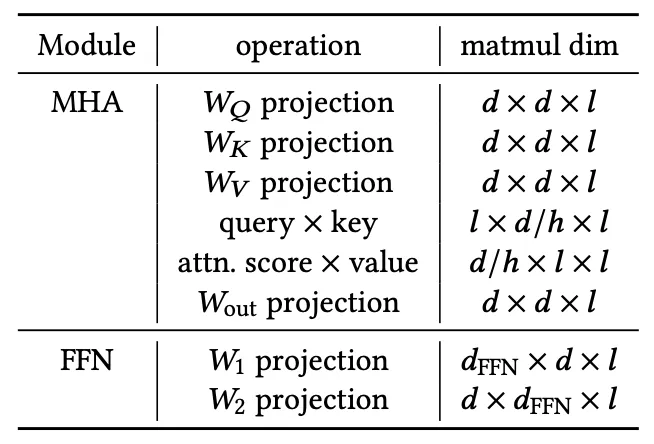Tab 1. Table from the paper “Full Stack Optimization of Transformer Inference: a Survey” showing the linear operations in Transformer models. The last column is the matrix multiplication dimensions, i.e., 𝑚 × 𝑛 × 𝑘 means the input dimensions of 𝑚 × 𝑛 and 𝑛 × 𝑘, and the output dimension of 𝑚 × 𝑘.

#### Nonlinear Operations

Nonlinear operations such as Softmax, LayerNorm, and GELU require specialized support and off-chip computation, which can be more challenging and incur significant overhead compared to linear operations when inferring with Transformer networks. These operations require multiple passes over all input values, which poses challenges in terms of efficient utilization of temporal memory and computation.

The Softmax operation involves exponential operations, summing up the results across the sequence length dimension, and normalizing the input by dividing it by the summation result.

Computing the LayerNorm function also requires multiple passes over the entire input values. The mean and standard deviation need to be computed and normalization to each input value applied. These nonlinear operations also pose challenges in operation fusing, a common technique to reduce interlayer communications by combining multiple operations into a single operation, which can lead to irregular tiling dimensions and lower data reuse.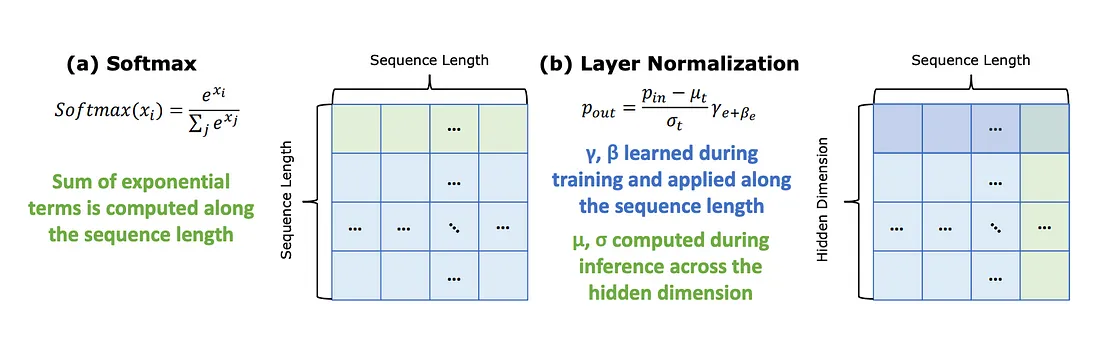Fig 2. Image from the paper “Full Stack Optimization of Transformer Inference: a Survey”. The diagrams outline the Softmax and LayerNorm operations. Since they rely on runtime statistics both require multiple passes over the input in order to compute the nonlinear operation.

#### Encoder and Decoder Architectures

The Transformer is an architecture introduced for machine translation tasks, consisting of encoder and decoder components. In subsequent works, encoder-only and decoder-only versions were introduced. The encoder block processes the input sequence in parallel and is suitable for natural language understanding tasks, while the decoder block processes tokens sequentially and is suitable for natural language generation tasks. The complexity of the encoder block scales linearly with sequence length, while the complexity of the decoder block scales linearly for projection layers and quadratically for act-to-act matmuls.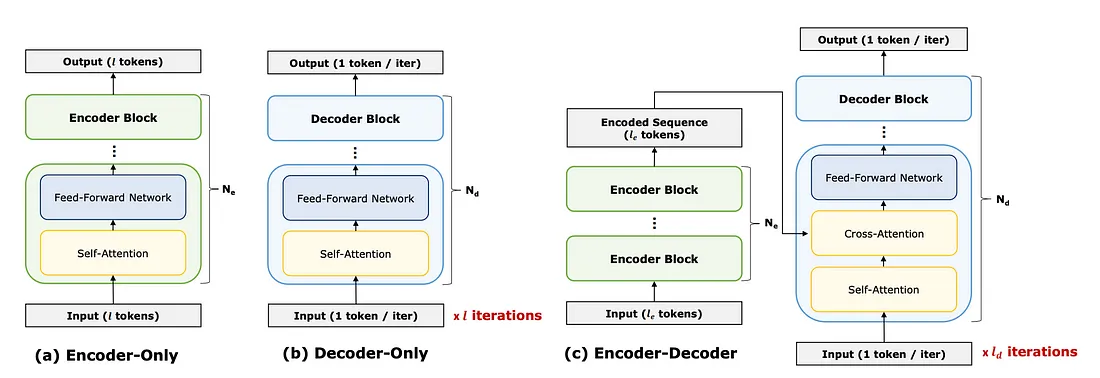Fig 3. Image from the paper “Full Stack Optimization of Transformer Inference: a Survey” showing different variants of Transformer networks. (a) An encoder-only model, which performs inference for all tokens in parallel. (b) A decoder-only model, which performs inference in an auto-regressive manner. (c) An encoder-decoder model uses the output of the encoded sequence as input to a cross-attention module.

## Model analysis

The authors evaluated bottlenecks in the Transformer architecture by modeling the number of floating-point operations (FLOPs) required to compute the Transformer encoder-only and decoder-only models, as well as the arithmetic intensity of these networks. The arithmetic intensity is the number of floating point operations that can be performed per byte loaded from memory, and it is computed by dividing the total number of FLOPs by the total number of bytes accessed (also referred to as MOPs, or memory operations).

The authors assumed that the local memories are large enough to hold both matrices entirely in memory for a given operation and counted the multiplication and addition from a MAC operation separately when computing FLOPs.

#### End-to-end FLOPs and MOPs

The study analyzed the encoder using 12-layer BERT-Base and 24-layer BERT-Large models, and the decoder using a 12-layer GPT-2 model. The authors ignored the maximum input sequence lengths of 512 for standard BERT models. They computed MOPs, the number of bytes that had to be accessed, and assumed 8-bit precision for all operations.

The FLOPs and MOPs for these models were plotted in the figures below and showed a super-linear scaling for all models, especially in the long sequence length regime due to the quadratic complexity with respect to sequence length in the act-to-act matmuls.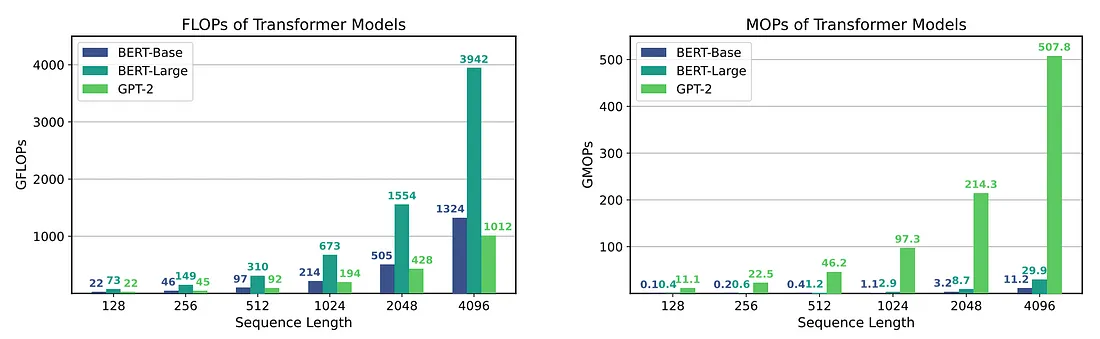Fig 4. Images from the paper “Full Stack Optimization of Transformer Inference: a Survey”. The image on the left shows the FLOPs for the BERT-Base and BERT-Large encoders and the GPT-2 decoder across different sequence lengths. The image on the right shows a plot of the MOPs for the BERT-Base and BERT-Large encoders and the GPT-2 decoder across different sequence lengths.

#### End-to-end Arithmetic Intensity

The arithmetic intensity was modeled by dividing the number of FLOPs required when inferring these models by the number of MOPs. For encoder-only BERT models, the arithmetic intensity initially increases with sequence length until 512 and then decreases afterward for larger sequence lengths due to the quadratic growth in the cost of act-to-act matmuls in the MHA module.

In contrast, the arithmetic intensity for decoder-only GPT-2 inference is significantly lower because it is composed solely of matrix-vector operations, which limits data reuse opportunities. It is important to note that GPT-2 has fewer FLOPs than BERT-Base and BERT-Large as the sequence length is increased. However, it is typically more challenging to run its inference efficiently due to its low arithmetic intensity.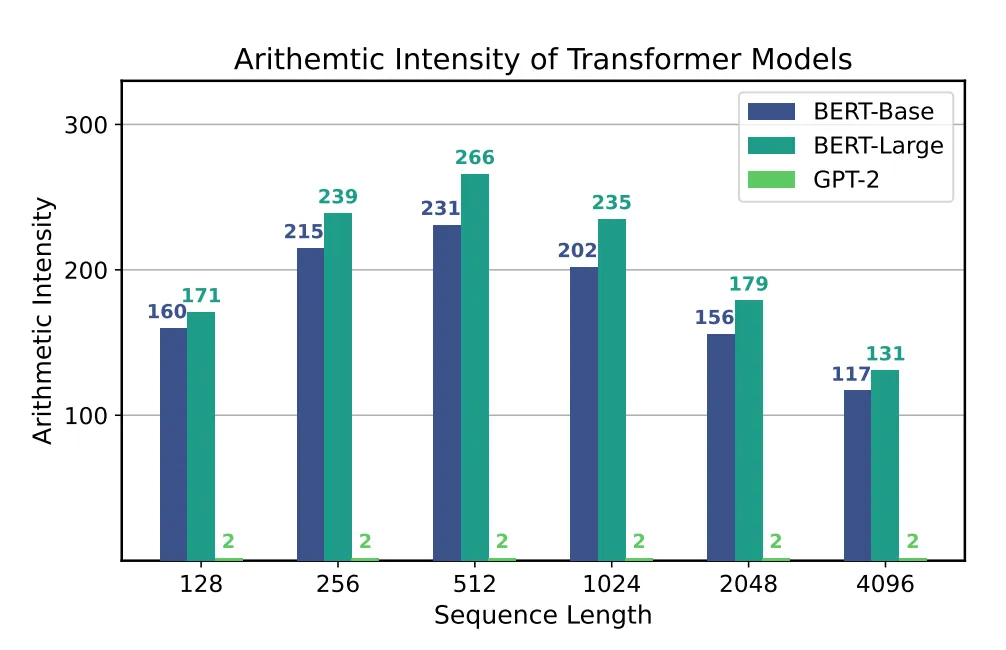Fig 5. Image from the paper “Full Stack Optimization of Transformer Inference: a Survey”. The plot shows how the arithmetic intensity of BERT-Base, BERT-Large, and GPT-2 changes with sequence length. Initially, the intensity increases but then decreases for larger sequence lengths due to the dominance of lower-intensity operations in the MHA module.

## Per-Layer FLOPs, MOPs, and Arithmetic Intensity

The per-layer FLOPs, MOPs, and arithmetic intensity of BERT-Base encoder were assessed with sequence length, and it was found that the proportion of FLOPs and MOPs consumed by act-to-act matmuls increases with sequence length. This decreases the overall arithmetic intensity of encoder-only models for long sequence lengths. The low arithmetic intensity of act-to-act matmuls is due to smaller matrix dimensions relative to other layers, leading to reduced reuse.

It is also noticed how the number of heads can impact the arithmetic intensity of the MHA module, in particular a hypothetical BERT model with a smaller number of heads would reduce the number of MOPs and improve the arithmetic intensity of the act-to-act attentions in the MHA module.

Nonlinear operations consume a significant proportion of MOPs, especially for longer sequence lengths.

The per-layer analysis of the GPT-2 decoder demonstrates the significantly reduced arithmetic intensity across all layers, compared to the encoder-only model, resulting from a large number of memory operations.

## Comparison with ResNet50

The article provides an analysis of ResNet50 as a baseline for comparison with Transformers. ResNet50 without any operator fusion has lower arithmetic intensity than BERT-Base across all sequence lengths. The low arithmetic intensity is partially due to the nonlinear operations in ResNet50 that consume a significant proportion of MOPs but can be fused with the preceding matmuls in a straightforward manner.

To optimize performance, ReLU operations can be directly applied to accumulated outputs, and BatchNorm operations can be integrated into previous convolutions. By fusing ReLU, the MOPs required for this operation are eliminated, and by folding BatchNorm, both the necessary FLOPs and MOPs are eliminated. Essentially, operation fusion is a method where the output values of one operation (such as a convolution or matmul) are used as input for the subsequent operation (such as ReLU or BatchNorm), without storing them in off-chip memory first. This eliminates the need for unnecessary memory loads and stores during nonlinear operations, leading to improved arithmetic intensity throughout the entire process.

Arithmetic intensity provides a rough estimate of how much data reuse is possible for different models and operations in the ideal case, analytical modeling can provide a more accurate estimate by taking account of hardware details.

## Profiling

To analyze the bottlenecks in Transformer workloads on commodity hardware, the authors profiled Transformer inference on an Intel Gold 6242 CPU. The workload latency was profiled breakdown for both encoder-only BERT-Base and decoder-only GPT-2.

#### Latency breakdown

The figures below show how the latency breakdown changes with respect to sequence length on a CPU for BERT-Base and GPT-2 models. The breakdowns demonstrate that for short sequence lengths, most of the computations are in the projection layers of the Feed-Forward Network (FFN) module and Multi-Head Attention (MHA) module, respectively. However, as sequence length increases, the act-to-act matrix multiplications (matmuls) become dominant as they scale quadratically with sequence length.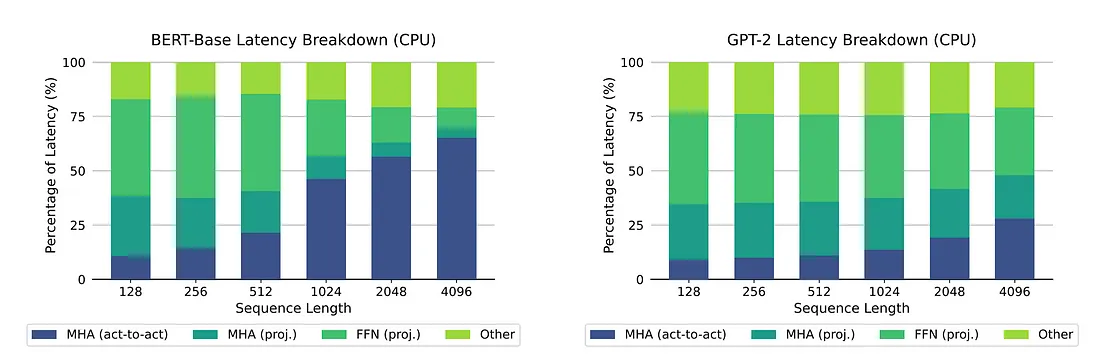Fig 6. Images taken from the paper “Full Stack Optimization of Transformer Inference: a Survey”. The image on the left shows how the computation breakdown in BERT-Base encoder changes with sequence length on a CPU. For shorter sequences, the projection layers dominate the model latency, but for longer sequences, the act-to-act matrix multiplications become dominant. The image on the right shows the computation breakdown in GPT-2 decoder with sequence length on a CPU. For shorter sequences, the projection layers dominate latency, but for longer sequences, the act-to-act matrix multiplications become significant. Nonlinear operations consume more latency than in encoder inference.

#### End-to-end Latency

The article discusses the normalized latency for BERT-Base, BERT-Large, and GPT-2 for different sequence lengths. Despite having similar configurations and end-to-end FLOPs, GPT-2 has a much longer latency than either BERT model due to its lower arithmetic intensity. This is mostly due to the lower arithmetic intensity of matrix-vector operations, and confirms that decoder inference is a memory-bound problem rather than a compute-bound problem. The article will revisit this issue to discuss existing methodologies for speeding up the decoding process.

## Hardware Design

So far the article analyzed the runtime characteristics and bottlenecks of Transformer architectures. In this section the paper focuses on full-stack solutions for efficient Transformer inference, starting with efficient hardware design.

#### Overview of Typical DNN Accelerators

A typical deep learning accelerator consists of:

• off-chip DRAM for holding the weights and activations
• on-chip global buffer memory (referred as global buffer) which needs to be large enough to hold a subset of the weights and inputs in order to feed the processing elements (PEs)
• an array of PEs, each capable of performing MAC operations, and which often contains one or more small local memories called register files (RFs)
• an internal network-on-chip (NoC) that transfers data between PEs

The local memories in PEs enable local data reuse to reduce global buffer accesses, which is crucial for optimizing energy efficiency. Without reuse, a MAC operation would require loading three parameters (the two input values that are being multiplied as well as the current partial sum) and storing the output value back to memory, which is significantly more expensive in terms of energy consumption.

There are two broad classes of dataflows that are widely adopted to maximize data reuse: temporal and spatial dataflows. DNN accelerators typically aim to leverage either temporal dataflows, by performing the same operation in parallel on several datapoints, or spatial dataflows, where data can be transferred between PEs to leverage additional reuse opportunities. Spatial dataflow reuse schemes include weight stationary dataflows, which hold weights in local memories in the PEs to improve reuse.

#### Hardware Design

Designing DNN accelerators for Transformers or adapting existing CNN accelerators requires consideration of several key factors. One of the main differences between the two is the optimal size for each level of the memory hierarchy and different memory bandwidth requirements due to differences in arithmetic intensity and matrix dimensions. Nonlinear functions present an additional challenge in hardware design, these operations require either specialized support for on-chip computation, or else they must be offloaded to the CPU. There are also considerations around datapath design, with specialized accelerators for the MHA module having less flexibility but better performance by reducing the number of required memory accesses. In contrast, accelerators for end-to-end Transformer inference are more flexible and use a similar structure to Gemmini. They perform individual operations separately in a matmul engine and aim to fuse operations for improved performance. However, the graph-level dataflow is not hardcoded in hardware as in MHA-specific accelerators.

Nonlinear function unit placement is important for optimizing arithmetic intensity through operator fusion in both MHA-specific and end-to-end Transformer accelerators. In MHA-specific accelerators, the Softmax unit must be placed in a way that enables operator-level fusion within the module. This requires careful consideration of the query × key and attention score × value multiplications. Placing functional units to support operator fusion can lead to higher efficiency but may sacrifice flexibility as the architecture assumes certain operator-level dataflow.

#### Analytical Modelling

This section discusses the use of analytic modelling as a tool for identifying performance bottlenecks in DNN benchmarks. Analytic modelling provides quick estimates of runtime behaviours on the target hardware platform and can guide design decisions in cases where profiling is difficult or infeasible. The authors developed an analytical model to demonstrate how it can be useful in understanding the performance breakdown of Transformer inference on hardware accelerators. The model assumes a Gemmini-driven architecture with local memories, a PE array, and an SFU for computing nonlinear operations, and assumes perfect overlap between compute time and memory operation time. Its structure is illustrated in the image below. The model also assumes a 𝑊-cycle latency for the PE array and 1-cycle latency per vector for the SFU.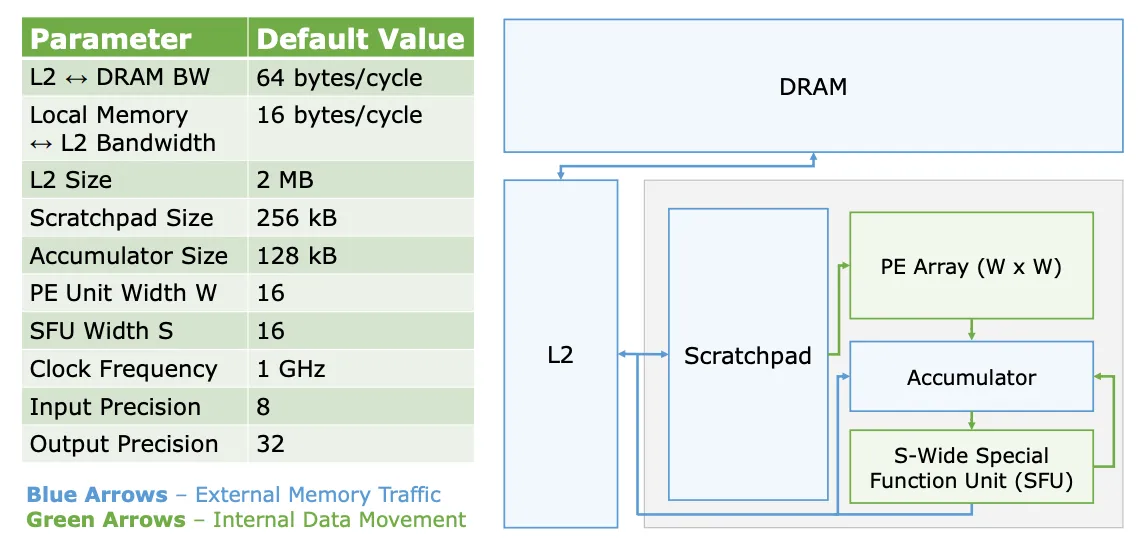Fig 7. Image taken from the paper “Full Stack Optimization of Transformer Inference: a Survey” showing the diagram of the structure of the basic analytical performance model, as well as the parameters that were varied in this analysis.

#### Overview of Typical DNN Accelerators

Analytic modeling can be used to estimate the latency breakdown and end-to-end runtime latency for models such as BERT-Base and BERT-Large encoders and the GPT-2 decoder. The modeling assumes square tiling and no operation fusion. The results of the analytic model show similar trends in runtime latency scaling and breakdowns as compared with the profiling results on the CPU. Note that the analytical model was designed assuming a hardware architecture that was different from the CPU architecture, and therefore the runtime behaviors would not necessarily be identical for different hardware platforms.

#### Non-ideal Arithmetic Intensity

The arithmetic intensity gives an estimate of data reuse in ideal scenarios, but real-world scenarios, like when tiling is required, can reduce the arithmetic intensity. Non-ideal arithmetic intensity, which takes into account hardware details, can provide a more accurate estimate in such cases.

The authors counted DRAM to L2 memory traffic in their analytical modeling to take the tiling effect into account, assuming 32-bit output precision before nonlinear operations. Non-ideal arithmetic intensities were provided for different operations in the BERT-Base encoder for sequence lengths of 128, 512, and 4096, which showed significant reductions compared to the ideal arithmetic intensity due to tiling and large 32-bit output activations. The reduction became even more pronounced for longer sequence lengths. This is in contrast to ResNet50, whose non-ideal arithmetic intensity was not significantly different from the ideal arithmetic intensity. Overall, the achieved arithmetic intensity for Transformers was lower than that of ResNet50, despite the ideal arithmetic intensity of Transformers being generally higher.

## Case Study: Building a Transformer Accelerator

The authors provide an example of how architects familiar with mainstream accelerators for convolutional, vision-based workloads can design state-of-the-art transformer accelerators. They start with the Gemmini accelerator-generator, which is optimized primarily for ResNet50-like workloads, and discuss the changes they made to it and its software stack to efficiently support transformer workloads such as BERT. They note that several accelerators for end-to-end transformer inference employ a similar structure to Gemmini and their analytical model, and also contain specialized post-processing units for nonlinear functions.

#### Baseline Accelerator

The article describes a baseline CNN accelerator generated by the Gemmini accelerator-generator. The accelerator uses a 16x16 systolic array to perform matmuls and convolutions, and has circuitry for ReLU and max-pool operations, as well as integer-float multipliers to scale 32-bit partial sums to 8-bit inputs that can be fed into the next layer in a CNN. However, it does not have any Transformer-specific features, such as support for non-linear normalization operations like LayerNorm, Softmax or support for the relatively expensive non-linear activation function GELU, which is often implemented with costly lookup tables. The baseline design is optimized for quantized integer CNN inference and achieves real-time or near-real-time performance on end-to-end CNN workloads, but performance on Transformer workloads such as BERT is limited.

#### Performance Bottlenecks

The baseline CNN accelerator generated by the Gemmini accelerator-generator is optimized for quantized integer CNN inference and achieves less than 1% utilization of its functional units when performing BERT inferences. While individual matmuls achieve a 74% utilization, operations not natively supported by the accelerator, such as LayerNorm, significantly decrease performance because they must be performed by the CPU instead. In fact, the image below shows that 96% of execution is spent on non-matmul operations.

The accelerator offloads GELU and Softmax operations to the host CPU, which consumes significantly more energy than the integer counterparts. Additionally, the memory hierarchy and memory bandwidth of the accelerator should be re-tuned for more efficient Transformer inference.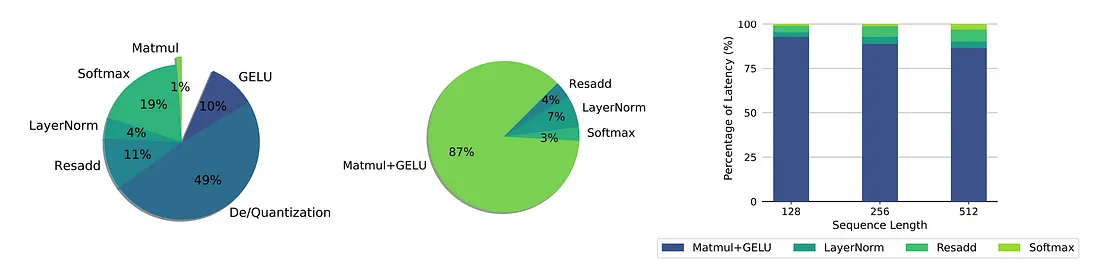Fig 8. Image taken from the paper “Full Stack Optimization of Transformer Inference: a Survey” showing the time spent on different operations during a BERT inference with a sequence-length of 512, when running on (Left) the baseline CNN accelerator, and (Middle) the accelerator after it has been extended with I-BERT’s hardware/software features for Transformers.

#### Memory Hierarchy

The shapes and arithmetic intensities of transformer matmuls are often different from those in convolutional layers in CNNs. Adjusting the sizes of the input/weight scratchpad and accumulator can significantly improve the performance of BERT’s matmul operations. Increasing the accumulation buffer size allows for improved output reuse, particularly with query x key matmuls. By reducing the shared input/weight scratchpad size and increasing the partial-sum accumulator size, there was a 36% reduction in total matmul latency with no increase in total SRAM capacity or total area of the accelerator.

#### Hardware-Software Co-Design

Matmuls are the dominant kernel in Transformer workloads, but the overhead of CPU-offloaded non-linear operations leads to low utilization. To alleviate this, a switch was made to an integer-only BERT variant called I-BERT, replacing floating-point non-linear operations (such as GELU and Softmax) with integer polynomial approximations for faster and cheaper implementation in specialized hardware accelerators. With new integer implementations of I-BERT’s GELU, LayerNorm, and Softmax variants added to the baseline CNN accelerator, overall end-to-end BERT inference performance improved by 39.6x with no need for quantization or dequantization. The new hardware units increased the total area consumption of the accelerator by only 14%, and the GELU, LayerNorm, and Softmax operations increased the power consumption of a BERT inference by only 9.3%. As Fig 8. illustrates, the computational bottleneck once again became the matmuls rather than normalization or activation functions, and the non-linear floating-point operations were replaced with integer polynomial approximations.

## Part 2 - Transformer Optmization

This is the end of the first part of the survey, in which we analyzed the bottlenecks and hardware implications of Transformers. In this second part of the survey, we will discuss Transformer optimization and neural architecture research.